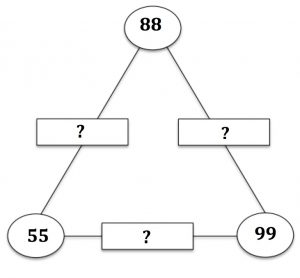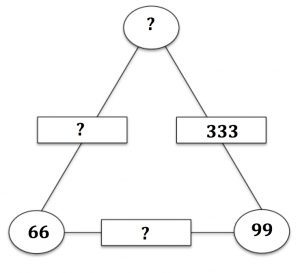The Checkit Game is suitable for all ages because you can choose the type of numbers and the operation that you will use.

You can play this game with (1) addition and subtraction or with (2) multiplication and division, whichever you choose.

The numbers on the edges of the triangle are the sum in Game 1 and the product in Game 2 of the numbers at the vertices.

So in this example Game 1 would have 143, 187 and 154 on the edges and Game 2 would have 4840, 8712 and 5445 on the edges.

Here are the rules for this game for 2 players:

1. The players must agree whether to play version (1) or version (2) of the game.
2. The players must agree on what numbers are allowed. For example whole numbers, or integers, or rational numbers, or powers of 2, 3, 4 and 7 or, to keep the numbers small, just 0 to 10 at the vertices.
3. Flip a coin to decide who goes first.
4. Players take it in turns to fill in a number in an empty box.
5. Players check if the numbers entered are correct. If a player makes a mistake and is challenged by an opponent before the opponent enters another number, then he loses the game and his opponent wins a point.
6. When all 6 boxes have numbers in them both players check and if there are any mistakes then both players lose a point, if everything is correct, both players win a point.
7. The first player to score 5 points wins the game.Here is another example for Game 1. What numbers go in the empty boxes? Click here for the answer.

What similarities and differences can you see between The Checkit Game with Addition and Polycircles https://aiminghigh.aimssec.ac.za/polycircles/ and The Triangle Midpoints Problem https://aiminghigh.aimssec.ac.za/triangle-midpoints/?